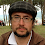## Wednesday, September 10, 2008

### Introducing `stable_vector`

We present `stable_vector`, a fully STL-compliant stable container that provides most of the features of `std::vector` except element contiguity. The underlying data structure has been discussed in a prior entry.

In the following we are using the terminology of the C++98 standard.

General properties. `stable_vector` satisfies all the requirements of a container, a reversible container and a sequence and provides all the optional operations present in `std::vector`. Like `std::vector`, iterators are random access. `stable_vector` does not provide element contiguity; in exchange for this absence, the container is stable, i.e. references and iterators to an element of a `stable_vector` remain valid as long as the element is not erased, and an iterator that has been assigned the return value of `end()` always remain valid until the destruction of the associated `stable_vector`.

Operation complexity. The big-O complexities of `stable_vector` operations match exactly those of `std::vector`. In general, insertion/deletion is constant time at the end of the sequence and linear elsewhere. Unlike `std::vector`, `stable_vector` does not internally perform any `value_type` destruction, copy or assignment operations other than those exactly corresponding to the insertion of new elements or deletion of stored elements, which can sometimes compensate in terms of performance for the extra burden of doing more pointer manipulation and an additional allocation per element.

Exception safety. (according to Abrahams' terminology) As `stable_vector` does not internally copy elements around, some operations provide stronger exception safety guarantees than in `std::vector`:

 operation exception safety for`std::vector` exception safety for`stable_vector` `insert` strong unless copy or assignment of `T` throw (basic) strong `erase` no-throw unless copy or assignment of `T` throw (basic) no-throw

Memory overhead. The C++ standard does not specifiy requirements on memory consumption, but virtually any implementation of `std::vector` has the same behavior wih respect to memory usage: the memory allocated by a vector `v` with n elements of type `T` is

mv = c·e,

where c is `v.capacity()` and e is `sizeof(T)`. c can be as low as n if the user has explicitly reserved the exact capacity required; otherwise, the average value c for a growing vector oscillates between 1.25·n and 1.5·n for typical resizing policies. For `stable_vector`, the memory usage is

msv = (c + 1)p + (n + 1)(e + p),

where p is the size of a pointer. We have c + 1 and n + 1 rather than c and n because a dummy node is needed at the end of the sequence. If we call f the capacity to size ratio c/n and assume that n is large enough, we have that

msv/mv ≈ (fp + e + p)/fe.

So, `stable_vector` uses less memory than `std::vector` only when e > p and the capacity to size ratio exceeds a given threshold:

msv < mvf > (e + p)/(ep). (provided e > p)

This threshold approaches typical values of f below 1.5 when e > 5p; in a 32-bit architecture, when e > 20 bytes.

Summary. `stable_vector` is a drop-in replacement for `std::vector` providing stability of references and iterators, in exchange for missing element contiguity and also some performance and memory overhead. When the element objects are expensive to move around, the performance overhead can turn into a net performance gain for `stable_vector` if many middle insertions or deletions are performed or if resizing is very frequent. Similarly, if the elements are large there are situations when the memory used by `stable_vector` can actually be less than required by `std::vector`.

1.mi pregunta es is your vector stable?

2.3.Well, I am currently maintaining a Boost library and in the process of integrating a new one, so I'm afraid I wouldn't have the time to cope with another library... But I wouldn't mind if someone else took the code and evolved it into a full-fledged proposal for Boost :)

4.This comment has been removed by the author.

5.If I try compiling stable_vector.hpp, I see this node_access element not declared error. Although I can see it is defined, even forward declaration does solve the issue, as we are using static function from node_access::get in the subsequent iterator class and it gives incomplete type error. I am using g++ 4.1.2 compiler. Am I missing something?

6.If I try compiling stable_vector.hpp, I see this node_access element not declared error. Although I can see it is defined, even forward declaration does solve the issue, as we are using static function from node_access::get in the subsequent iterator class and it gives incomplete type error. I am using g++ 4.1.2 compiler. Am I missing something?

This looks like a compiler bug, will try to have a look at it when I have access to a g++ 4.1.x installation. Meanwhile, please try moving the definition of node_access just before that of iterator (currently it's just after iterator). Does this solve things?

7.I tried even moving definition of node_access before iterator class, but again it gives error for iterator: expected nested-name-specifier.
Ah, I guess, now I can put in the forward declaration of iterator class before node_access. That should solve the problem. Let me try that.

8.Yes, moving definition and iterator forward declaration helped. So I guess, 4.1.2 compiler has a bug, as also tried with 3.4.4 and it worked without any change.

Thanks.

9.What is the difference between stable_vector and std::deque?

10.What is the difference between stable_vector and std::deque?

std::deque provides constant time insertion both at the beginning and the end of the sequence (stable_vector and std::vector only at the end). std::queue does so at the expense of a more costly data structure. You might want to take a look at the article "Profiling stable_vector" to see the various tradeoffs involved